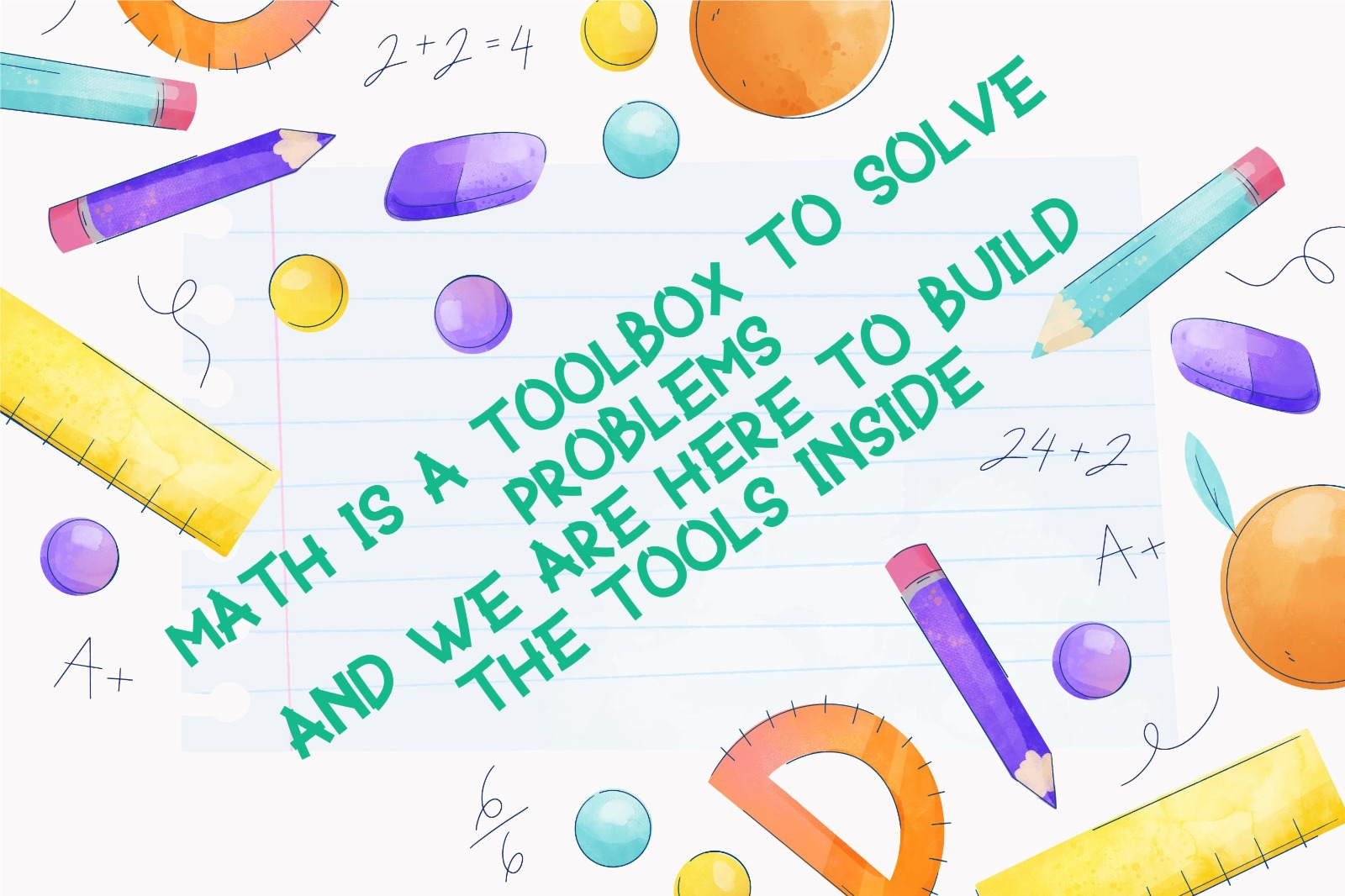Mathematic Tutor – Michelle Tsai

• University of Minnesota Twin Cities MATHEMATICS BA, major PURE MATHEMATICS
• Experienced in both Hong Kong and US Exam and Education Systems
• Expert in delivering Math in interesting ways

### My Teaching Philosophy:

“What is the point of studying math when we would not use it in real life?” When I was a student, I always had that question in my mind. So, why is math so important? And why do we have to know math?

As a previous troublemaker, I can relate why students fear math, or even hate math. Students might think math is only for exams, however, everything in life is related to math. From getting a bag of chips to calculating the calorie intake, math applies to all daily activities. All these life experiences will convert to the word problems on the math exam, and we are here to master this tool to solve all problems.

In our daily lives, we come across different math problems. Like getting groceries, we might think (calculate) how we could get the best discount, or what we need at home. We would like to get the best price with the right amount that we need. Then we need to do some calculations. There are so many more problems such as bank interest, racing, floor plan, etc. All these relate to math topics like percentage, probabilities, space and measuring. Then, we need the math skills from the toolbox.Now we know why we need math, let’s talk about how we can explore math. There is only one set of solutions for each math problem, but there is no limit on how we find the answer. As a tutor, I like to explain math with different examples. I like to explore with the students what methods would work best for them, and what methods require the least number of steps. By finding the most suitable tool, we can easily solve the problems in a short amount of time, like during the exams.

I am confident that every student here would succeed in math after knowing the importance of math and getting their own methods(tools).